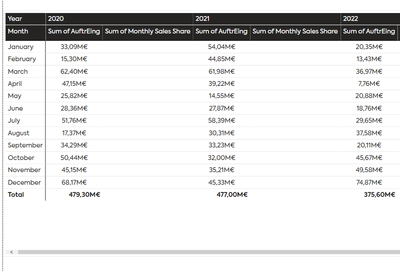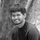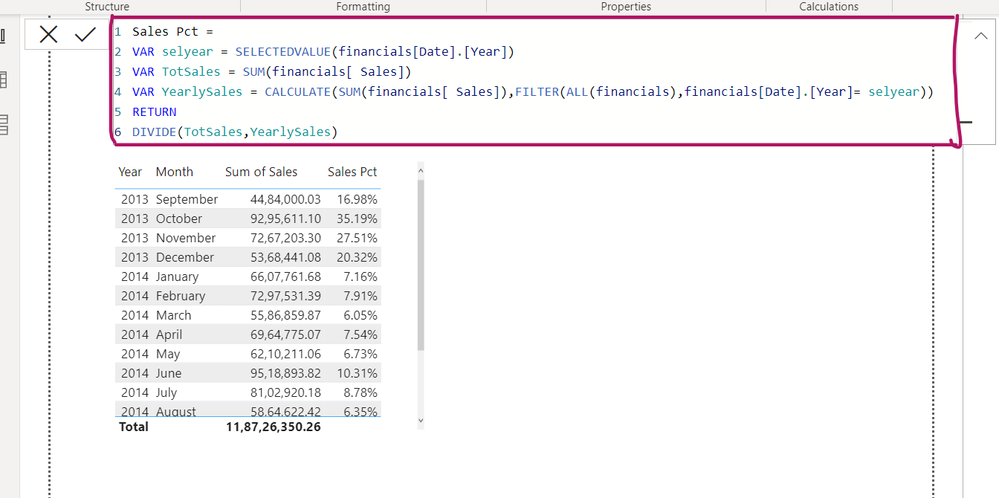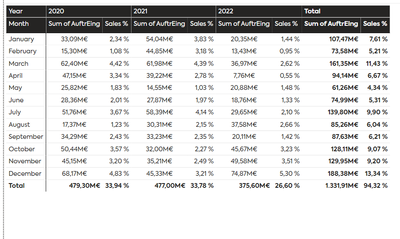cancel
Showing results for
Did you mean:Helper II

## % share of the total sales for each month

Hello,

I want to calculate the % share of each months sale over the total sale for each year in the following visual:So for example the total sales for the year 2020 was 479.39 M.
Hence January months' share towards the total sale in 2020 would be : 6.90%.

Can someone help me?

1 ACCEPTED SOLUTIONSuper User

add that category column in the allexcept, check the below

``````Sales % =
VAR _sumOfSales = SUM(Sales[Sales])
VAR _totalSales = CALCULATE(
SUM(financials[ Sales]),ALLEXCEPT(financials,financials[Year],financials[Segment]))
VAR _result = DIVIDE([Sum of Sales],_totalSales)
RETURN _result``````

Thanks,

Arul

Regards,
Arul
If I answered your question kindly mark my post as a solution and a kudo would be appreciated.
6 REPLIES 6Solution Sage

Hope this helps:Super User

modify this based on your need and try,

``````Sales % =
VAR _sumOfSales = SUM(Sales[Sales])
VAR _totalSales = CALCULATE(
SUM(financials[ Sales]),ALL(financials))
VAR _result = DIVIDE([Sum of Sales],_totalSales)
RETURN _result``````

Thanks,

Arul

Regards,
Arul
If I answered your question kindly mark my post as a solution and a kudo would be appreciated.Helper II

Hello,

Thanks for your reply. But I am not getting the correct output with this measure.For example the % share for January 2020 should have been 6.9% but its coming to be 2.34% with your measure.

Sales % =
VAR _sumOfSales = SUM('MyTable'[AuftrEing])
VAR _totalSales = CALCULATE(
SUM('MyTable'[AuftrEing]),ALL('MyTable'))
VAR _result = DIVIDE(_sumOfSales,_totalSales)
RETURN _resultSuper User

there is a small changes in the formula. Can you modify and try this formula now?

)

``````Sales % =
VAR _sumOfSales = SUM(Sales[Sales])
VAR _totalSales = CALCULATE(
SUM(financials[ Sales]),ALLEXCEPT(financials,financials[Year]))
VAR _result = DIVIDE([Sum of Sales],_totalSales)
RETURN _result``````

Thanks,

Arul

Regards,
Arul
If I answered your question kindly mark my post as a solution and a kudo would be appreciated.Helper II

Hello @Arul

Thanks again for your solution its working only with one exception. I have a page level categorical filter. And when I select a category in that filter it doesn't gets applied to the measure. How to handle that?Super User

add that category column in the allexcept, check the below

``````Sales % =
VAR _sumOfSales = SUM(Sales[Sales])
VAR _totalSales = CALCULATE(
SUM(financials[ Sales]),ALLEXCEPT(financials,financials[Year],financials[Segment]))
VAR _result = DIVIDE([Sum of Sales],_totalSales)
RETURN _result``````

Thanks,

Arul

Regards,
Arul
If I answered your question kindly mark my post as a solution and a kudo would be appreciated.Announcements#### Exclusive opportunity for Women!

Join us for a free, hands-on Microsoft workshop led by women trainers for women where you will learn how to build a Dashboard in a Day!#### Power Platform Conference-Power BI and Fabric Sessions

Join us Oct 1 - 6 in Las Vegas for the Microsoft Power Platform Conference.Top Solution Authors
Top Kudoed Authors
Users online (2,085)